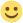# Repaint Tutorial (now with Colour-Code Wireframe) - How To Repaint Fernbus

Welcome to the new TML-Studios Community! Please read through the Community Rules and Guidelines and Tips & Tricks. Have fun!
• Hi there,

I thought a tutorial showing you how to make your own repaint may be of use, so here it is.

Content embedded from external sources will not be displayed without your consent.
Through the activation of external content, you agree that personal data may be transferred to third party platforms. We have provided more information on this in our privacy policy.

I hope it helps+=+=+=+=+=+=+=+=+=+=+=+=+=+=+=+=+=+=+=+=+=+=+=+=+=+=+=+=+=+=+=+=+=+=+=+=+=+=+=+=+=+=+=+=+=+=+=+=+=+=+=+=+=+=+=+=+=+=+=+=+=+=+=

UPDATE

I hope it helpsIn an attempt to make repaints easier I have created a colour-coded wireframe see here:

If it's not useful then OK no worries